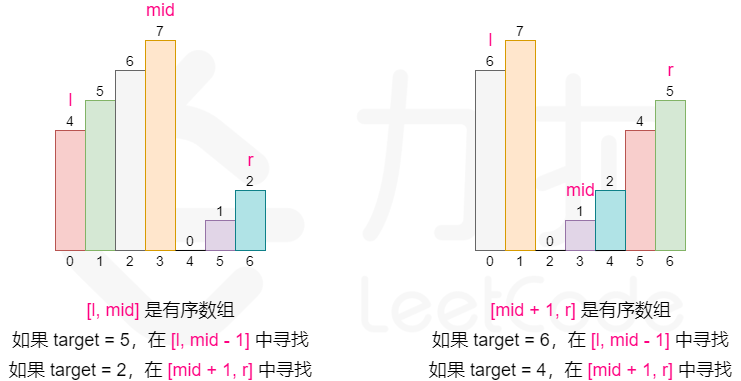# 33. 搜索旋转排序数组 Search in Rotated Sorted Array

## 题目

### 例子

输入：nums = [4,5,6,7,0,1,2], target = 0



输入：nums = [4,5,6,7,0,1,2], target = 3



输入：nums = , target = 0



1 <= nums.length <= 5000

-10^4 <= nums[i] <= 10^4

nums 中的每个值都 独一无二

-10^4 <= target <= 10^4

## v1-二分法青春版

### 思路

[0,1,2,4,5,6,7] 在下标 3 处经旋转后可能变为 [4,5,6,7,0,1,2] 。

### 实现

java
/**
* Input: nums = [4,5,6,7,0,1,2], target = 0
* Output: 4
* @param nums
* @param target
* @return
*/
public int search(int[] nums, int target) {
// 没有旋转，或者全部旋转
int randomK = getRandomK(nums);
if(-1 == randomK) {
return binarySearch(nums, target, 0, nums.length-1);
}

// 将数组拆成2个部分
// 4 5 6 7 0 1 2 => [4 5 6 7] [0 1 2]
// 3 1 ==>  
int leftIndex = binarySearch(nums, target, 0, randomK);
if(leftIndex != -1) {
return leftIndex;
}

// 右边寻找
int rightIndex = binarySearch(nums, target, randomK+1, nums.length-1);
if(rightIndex != -1) {
return rightIndex;
}

// 如果不存在
return -1;
}

/**
* 获取随机数
*
* 寻找 k > k+i 的位置
*
* [4,5,6,7,0,1,2]
* @param nums 数组
* @return 变化的长度
* @since v33
*/
private int getRandomK(final int[] nums) {
for(int i = 0; i < nums.length-1; i++) {
if(nums[i] > nums[i+1]) {
return i;
}
}

// 根据顺序找到即可
return -1;
}

/**
* 二分查询
* <p>
* 备注：ASC
*
* @param nums   原始数组
* @param target 目标值
* @return 结果
* @since v33
*/
private static int binarySearch(int[] nums, int target, int low, int high) {
while (low <= high) {
int mid = (high+low)/2;
int midVal = nums[mid];

// 刚好相等
if (target == midVal) {
return mid;
} else if (target > midVal) {
// 当前信息偏小
low = mid+1;
} else {
// 数据偏大
high = mid-1;
}
}

return -1;
}


## v2-二分法

### 思路### java 实现

    public int search(int[] nums, int target) {
int n = nums.length;
if (n == 0) {
return -1;
}
if (n == 1) {
return nums == target ? 0 : -1;
}
int l = 0, r = n - 1;
while (l <= r) {
int mid = (l + r) / 2;
if (nums[mid] == target) {
return mid;
}
if (nums <= nums[mid]) {
if (nums <= target && target < nums[mid]) {
r = mid - 1;
} else {
l = mid + 1;
}
} else {
if (nums[mid] < target && target <= nums[n - 1]) {
l = mid + 1;
} else {
r = mid - 1;
}
}
}
return -1;
}


# 81. 搜索旋转排序数组 II Search in Rotated Sorted Array II

## 题目

### 例子

输入：nums = [2,5,6,0,0,1,2], target = 0



输入：nums = [2,5,6,0,0,1,2], target = 3



## 思路

## java 实现

java
public boolean search(int[] nums, int target) {
int n = nums.length;
if (n == 0) {
return false;
}
if (n == 1) {
return nums == target;
}
int l = 0, r = n - 1;
while (l <= r) {
int mid = (l + r) / 2;
if (nums[mid] == target) {
return true;
}

// 处理一下
if (nums[l] == nums[mid] && nums[mid] == nums[r]) {
++l;
--r;
} else if (nums[l] <= nums[mid]) {
if (nums[l] <= target && target < nums[mid]) {
r = mid - 1;
} else {
l = mid + 1;
}
} else {
if (nums[mid] < target && target <= nums[n - 1]) {
l = mid + 1;
} else {
r = mid - 1;
}
}
}
return false;
}


# 153. 寻找旋转排序数组中的最小值

## 题目

### 例子

输入：nums = [3,4,5,1,2]



输入：nums = [4,5,6,7,0,1,2]



输入：nums = [11,13,15,17]



n == nums.length

1 <= n <= 5000

-5000 <= nums[i] <= 5000

nums 中的所有整数 互不相同

nums 原来是一个升序排序的数组，并进行了 1 至 n 次旋转

## 思路



The minimum element must satisfy one of two conditions:

[4,5,6,7,0,1,2]

1) If rotate, A[min] < A[min - 1];

2) If not, A.

Therefore, we can use binary search: check the middle element, if it is less than previous one, then it is minimum.

If not, there are 2 conditions as well: If it is greater than both left and right element, then minimum element should be on its right, otherwise on its left.


## java 实现

java
public int findMin(int[] nums) {
int start = 0;
int end = nums.length - 1;
while (start < end) {
int mid = (start +end) / 2;

// 如果是旋转的场景。不旋转的话，一定大于前面
// [4,5,6,7,0,1,2]
if(mid > 0 &&
nums[mid] < nums[mid-1]) {
return nums[mid];
}

// 如果当前元素比2边都大，那就是右边。
if(nums[mid] >= nums[start]
&& nums[mid] >= nums[end]) {
start = mid+1;
} else {
end = mid-1;
}
}

return nums[start];
}


# 154. 寻找旋转排序数组中的最小值 II

## 思路

0 1 2 3 4

1）默认情况下，如果 nums[lo] < nums[hi] 那么我们返回 nums[lo] 因为数组从未旋转过，或者旋转过 n 次。

2）进入while循环后，我们检查

if nums[mid] > nums[hi] => lo = mid + 1 因为最小元素在数组的右半部分

2 3 4 0 1

else if nums[mid] < nums[hi] => hi = mid 因为最小元素在数组的左半部分

7 0 1 2 3 4 5 6

else => hi-- 处理重复值

## java 实现

public int findMin(int[] nums) {
int low = 0, high = nums.length-1;
// default
if(nums[low] < nums[high]) {
return nums[low];
}

while (low < high) {
int mid = (low + high) / 2;
//1. 大于最大,在右边
if(nums[mid] > nums[high]) {
low = mid+1;
} else if(nums[mid] < nums[high]) {
//2. 小于最大，则在左边
high = mid;
} else {
// 重复
high--;
}
}
return nums[high];
}


# 开源地址

https://github.com/houbb/leetcode

# 参考资料

https://leetcode.cn/problems/search-in-rotated-sorted-array/

https://leetcode.cn/problems/find-minimum-in-rotated-sorted-array/